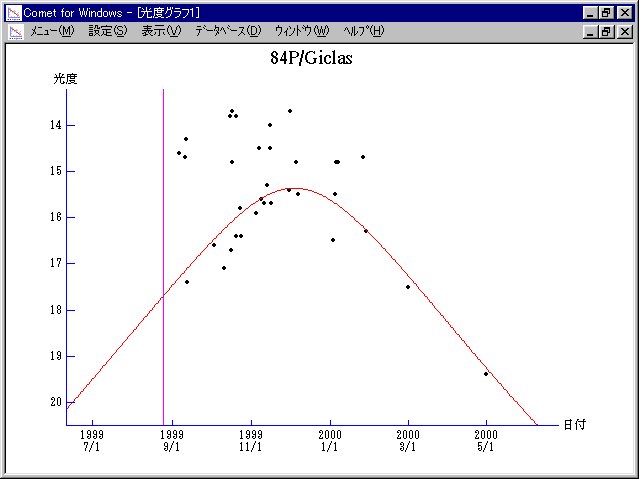# \$B%.%/%i%9WB@1(B

84P/Giclas (1999)

 English version Home page Updated on September 29, 2004###\$B###\$B50F;MWAG(B

```   The following improved orbital elements by Kenji Muraoka, are
from 244 observations 1931 to 2000, perturbations by 9 Plantes,
Moon and 5 minor planets were taken into account.
The mean residual is +/- 0.94 arc seconds.

Epoch  =  1999 Aug. 10.0  TT       JDT = 2451400.5
T  =  1999 Aug. 25.13062       +/- 0.00014 (m.e.) TT
Peri. =  276.41786                +/- 0.00014
Node  =  112.48959                +/- 0.00014   (2000.0)
Incl. =    7.28163                +/- 0.00001
q  =    1.8458518              +/- 0.0000005 AU
e  =    0.4936352              +/- 0.0000001
a  =    3.6453002              +/- 0.0000004 AU
n  =    0.14161333             +/- 0.00000002
P  =    6.960                  +/- 0.0000012  years
```

###\$B@1?^(B###\$B8wEYJQ2=(B

```        m1 = 8.5 + 5 log\$B&\$(B + 25 log r(t - 123)
```##### \$B50F;MWAG\$OB<2,7r<#;a\$N7W;;\$K\$h\$k\$b\$N\$G\$9!#(B \$B@1?^\$O(B StellaNavigator Ver.2.0 for Windows (\$B%"%9%H%m%"!<%D(B \$BJTCx(B / \$B%"%9%-!<=PHG6I4)(B) \$B\$G:n@.\$7\$?\$b\$N\$G\$9!#(B \$B8wEY%0%i%U\$O(BComet for Windows\$B\$G:n@.\$7\$?\$b\$N\$G\$9!#(B